Courses

# Entropy Electronics and Communication Engineering (ECE) Notes | EduRev

## Communication Theory

Created by: Machine Experts

## Electronics and Communication Engineering (ECE) : Entropy Electronics and Communication Engineering (ECE) Notes | EduRev

The document Entropy Electronics and Communication Engineering (ECE) Notes | EduRev is a part of the Electronics and Communication Engineering (ECE) Course Communication Theory.
All you need of Electronics and Communication Engineering (ECE) at this link: Electronics and Communication Engineering (ECE)

Entropy: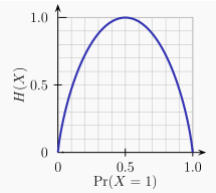Entropy of a Bernoulli trial as a function of success probability, often called the binary entropy function, Hb(p). The entropy is maximized at 1 bit per trial when the two possible outcomes are equally probable, as in an unbiased coin toss. The entropy, H, of a discrete random variable X is a measure of the amount of uncertainty associated with the value of X.

Suppose one transmits 1000 bits (0s and 1s). If these bits are known ahead of transmission (to be a certain value with absolute probability), logic dictates that no information has been transmitted. If, however, each is equally and independently likely to be 0 or 1, 1000 bits (in the information theoretic sense) have been transmitted. Between these two extremes, information can be quantified as follows. If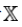is the set of all messages{x1,...,xn} that X could be, and p(x) is the probability of X given some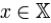, then the entropy of X is defined: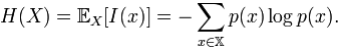(Here, I(x) is the self-information, which is the entropy contribution of an individual message, and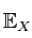is the expected value.) An important property of entropy is that it is maximized when all the messages in the message space are equiprobable p(x) = 1 / n,—i.e., most unpredictable—in which case H(X) = logn.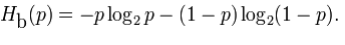Joint entropy:

The joint entropy of two discrete random variables X and Y is merely the entropy of their pairing: (X,Y). This implies that if X and Y are independent, then their joint entropy is the sum of their individual entropies.

For example, if (X,Y) represents the position of a chess piece - X the row and Y the column, then the joint entropy of the row of the piece and the column of the piece will be the entropy of the position of the piece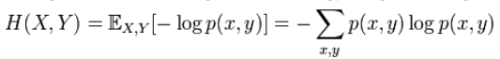Despite similar notation, joint entropy should not be confused with cross entropy.

Conditional entropy (equivocation):

The conditional entropy or conditional uncertainty of X given random variable Y (also called the equivocation of X about Y) is the average conditional entropy over Y: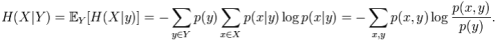Because entropy can be conditioned on a random variable or on that random variable being a certain value, care should be taken not to confuse these two definitions of conditional entropy, the former of which is in more common use. A basic property of this form of conditional entropy is that: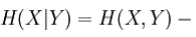H (Y)

Mutual information (transinformation):

Mutual information measures the amount of information that can be obtained about one random variable by observing another. It is important in communication where it can be used to maximize the amount of information shared between sent and received signals. The mutual information of X relative to Y is given by: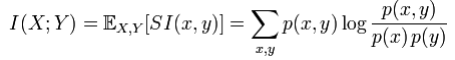where SI (Specific mutual Information) is the pointwise mutual information.

A basic property of the mutual information is that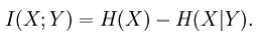That is, knowing Y, we can save an average of I(X;Y) bits in encoding X compared to not knowing Y.
Mutual information is symmetric: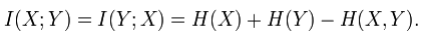Mutual information can be expressed as the average Kullback – Leibler divergence (information gain) of the posterior probability distribution of X given the value of Y to the prior distribution on X: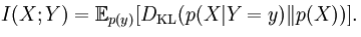In other words, this is a measure of how much, on the average, the probability distribution on X will change if we are given the value of Y. This is often recalculated as the divergence from the product of the marginal distributions to the actual joint distribution: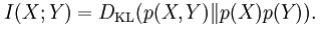Mutual information is closely related to the log-likelihood ratio test in the context of contingency tables and the multinomial distribution and to Pearson's χ2 test: mutual information can be considered a statistic for assessing independence between a pair of variables, and has a well-specified asymptotic distribution.

Kullback–Leibler divergence (information gain):

The Kullback–Leibler divergence (or information divergence, information gain, or relative entropy) is a way of comparing two distributions: a "true" probability distribution p(X), and an arbitrary probability distribution q(X). If we compress data in a manner that assumes q(X) is the distribution underlying some data, when, in reality, p(X) is the correct distribution, the Kullback–Leibler divergence is the number of average additional bits per datum necessary for compression. It is thus defined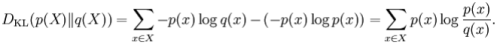Although it is sometimes used as a 'distance metric', it is not a true metric since it is not symmetric and does not satisfy the triangle inequality (making it a semi-quasimetric).

Coding theory:

Coding theory is one of the most important and direct applications of information theory. It can be subdivided into source coding theory and channel coding theory. Using a statistical description for data, information theory quantifies the number of bits needed to describe the data, which is the information entropy of the source.

Data compression (source coding): There are two formulations for the compression problem:

1. lossless data compression: the data must be reconstructed exactly;
2. lossy data compression: allocates bits needed to reconstruct the data, within a specified fidelity level measured by a distortion function. This subset of Information theory is called rate–distortion theory.
• Error-correcting codes (channel coding): While data compression removes as much redundancy as possible, an error correcting code adds just the right kind of redundancy (i.e., error correction) needed to transmit the data efficiently and faithfully across a noisy channel.

This division of coding theory into compression and transmission is justified by the information transmission theorems, or source–channel separation theorems that justify the use of bits as the universal currency for information in many contexts. However, these theorems only hold in the situation where one transmitting user wishes to communicate to one receiving user. In scenarios with more than one transmitter (the multiple-access channel), more than one receiver (the broadcast channel) or intermediary "helpers" (the relay channel), or more general networks, compression followed by transmission may no longer be optimal. Network information theory refers to these multi-agent communication models.

SOURCE THEORY:

Any process that generates successive messages can be considered a source of information. A memoryless source is one in which each message is an independent identically-distributed random variable, whereas the properties of ergodicity and stationarity impose more general constraints. All such sources are stochastic. These terms are well studied in their own right outside information theory.

Rate: Information rate is the average entropy per symbol. For memoryless sources, this is merely the entropy of each symbol, while, in the case of a stationary stochastic process, it is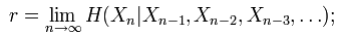that is, the conditional entropy of a symbol given all the previous symbols generated. For the more general case of a process that is not necessarily stationary, the average rate is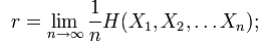that is, the limit of the joint entropy per symbol. For stationary sources, these two expressions give the same result. www.VidyarthiPlus.in www.VidyarthiPlus.in It is common in information theory to speak of the "rate" or "entropy" of a language. This is appropriate, for example, when the source of information is English prose. The rate of a source of information is related to its redundancy and how well it can be compressed, the subject of source coding.

Channel capacity:

Communications over a channel—such as an ethernet cable—is the primary motivation of information theory. As anyone who's ever used a telephone (mobile or landline) knows, however, such channels often fail to produce exact reconstruction of a signal; noise, periods of silence, and other forms of signal corruption often degrade quality. How much information can one hope to communicate over a noisy (or otherwise imperfect) channel? Consider the communications process over a discrete channel. A simple model of the process is shown below: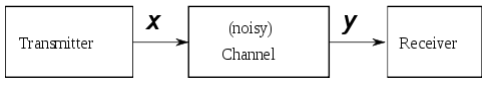Here X represents the space of messages transmitted, and Y the space of messages received during a unit time over our channel. Let p(y | x) be the conditional probability distribution function of Y given X. We will consider p(y | x) to be an inherent fixed property of our communications channel (representing the nature of the noise of our channel). Then the joint distribution of X and Y is completely determined by our channel and by our choice of f(x), the marginal distribution of messages we choose to send over the channel. Under these constraints, we would like to maximize the rate of information, or the signal, we can communicate over the channel. The appropriate measure for this is the mutual information, and this maximum mutual information is called the channel capacity and is given by: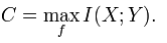This capacity has the following property related to communicating at information rate R (where R is usually bits per symbol). For any information rate R < C and coding error ε > 0, for large enough N, there exists a code of length N and rate ≥ R and a decoding algorithm, such that the maximal probability of block error is ≤ ε; that is, it is always possible to transmit with arbitrarily small block error. In addition, for any rate R > C, it is impossible to transmit with arbitrarily small block error.

Channel coding is concerned with finding such nearly optimal codes that can be used to transmit data over a noisy channel with a small coding error at a rate near the channel capacity.

Bit Rate

In telecommunications and computing, bitrate (sometimes written bit rate, data rate or as a variable R or fb) is the number of bits that are conveyed or processed per unit of time.

The bit rate is quantified using the bits per second (bit/s or bps) unit, often in conjunction with an SI prefix such as kilo- (kbit/s or kbps), mega-(Mbit/s or Mbps), giga- (Gbit/s or Gbps) or tera- (Tbit/s or Tbps). Note that, unlike many other computer-related units, 1 kbit/s is traditionally defined as 1,000 bit/s, not 1,024 bit/s, etc., also before 1999 when SI prefixes were introduced for units of information in the standard IEC 60027-2.

The formal abbreviation for "bits per second" is "bit/s" (not "bits/s", see writing style for SI units). In less formal contexts the abbreviations "b/s" or "bps" are often used, though this risks confusion with "bytes per second" ("B/s", "Bps"). 1 Byte/s (Bps or B/s) corresponds to 8 bit/s (bps or b/s).

Offer running on EduRev: Apply code STAYHOME200 to get INR 200 off on our premium plan EduRev Infinity!

,

,

,

,

,

,

,

,

,

,

,

,

,

,

,

,

,

,

,

,

,

;